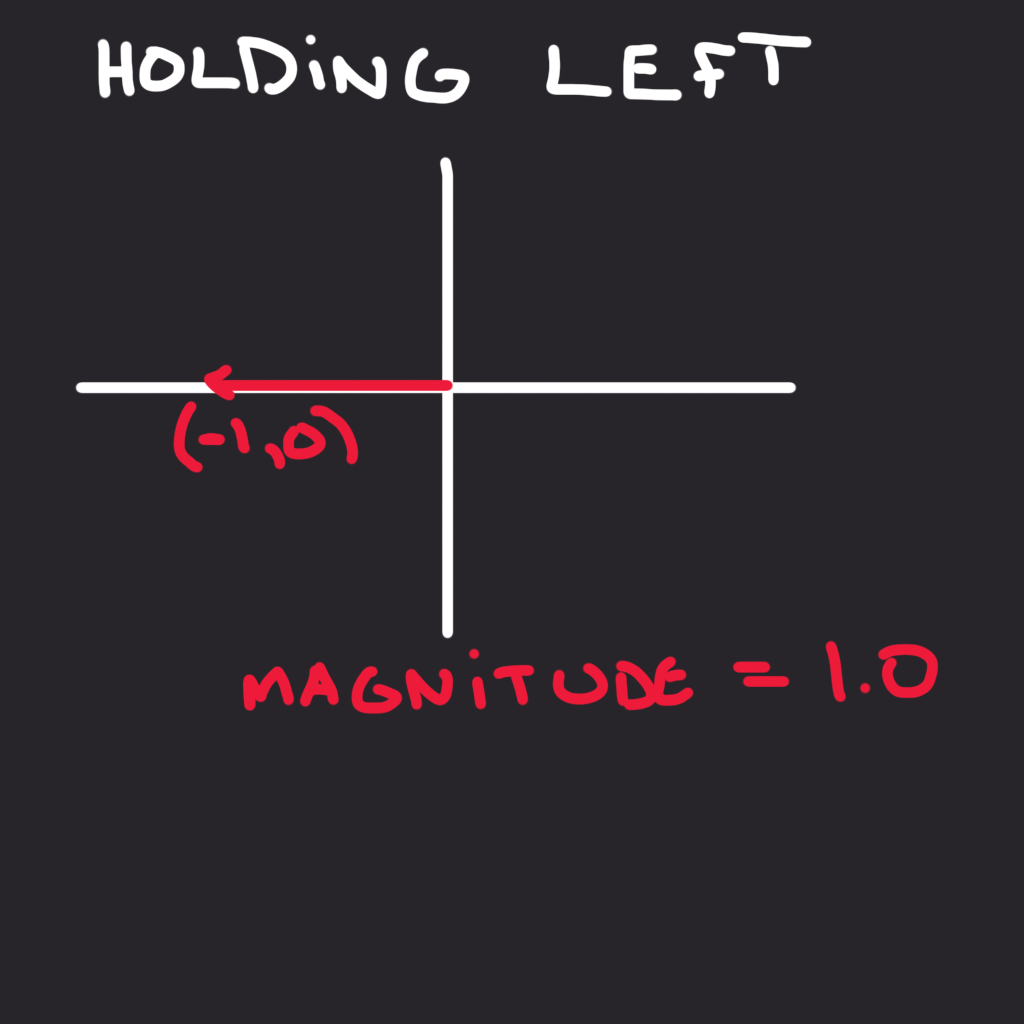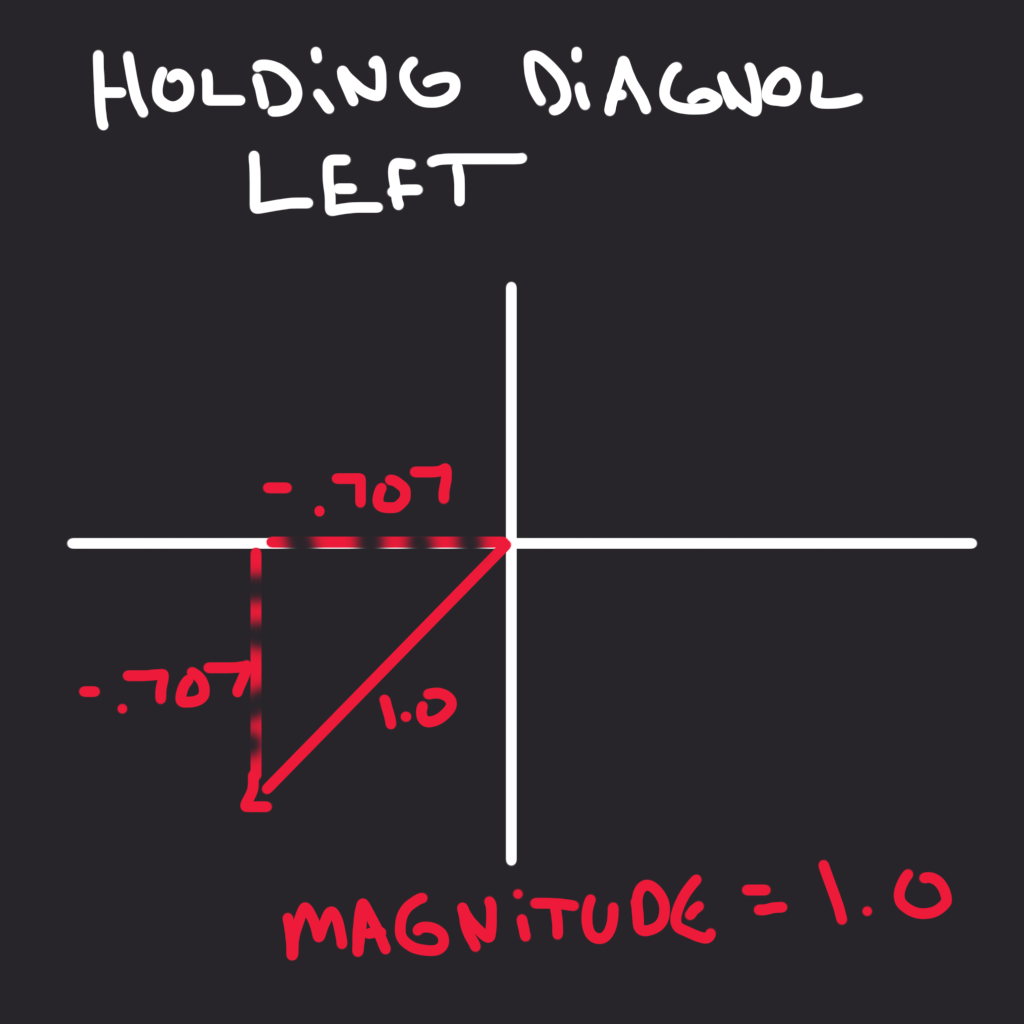RichardO

# using if statement

different between the if direction.length() > 1.0 and the if direction:

i also try the if statement without the .length > 1.0 just the if direction: only dont understand why it do the same

•RichardO replied
and it is possible to reset all the code so i can write it from scratc
• a
andrewjhaugen replied
Solution

The if direction.length()> 1.0 is there to insure that we don't move faster diagonally than we do horizontally. If we didn't check for the length of the direction to be bigger than 1, than the ship would actually move faster when moving diagonally than moving horizontally. By normalizing, we get the behavior I am showing in the pictures where we get a consistent magnitude (or length) of 1.01 love
•RichardO replied

Actually i already know that. my problem is the if statement code.

here.

if direction.length() > 1.0:

and the

if direction:

dont understand why in the code they do this. i think they do the same thing

•Nathan Lovato replied

It's not the same conditional check. In the first case, as Andrew mentioned, the condition is true only when the ship is moving diagonally. We only want to normalize the vector if it's too long, because there are cases where the length of the direction vector can be less than 1 (when lightly pushing a joystick), and normalizing the vector in those cases would make the ship move at full speed instead of letting the player control more finely with the joystick.

On the other hand, if direction only checks if the direction vector is not Vector2(0, 0) or if the value isn't null. So it checks if the player is trying to move in any direction, even horizontally, even when lightly pushing the joystick. In those cases, we want to rotate the ship's sprite to match the player's input direction.

But we don't want to change the rotation when there's no direction vector, otherwise, the ship would snap back to looking to the right upon releasing all movement keys.

1 love
• M
Mikimic replied

If this is still a question - here is my understanding of it.

1. if direction > 1:

This direction > 1 checks that the magnitude (length) of the vector (in our case the vector is called "direction"). Think of a vector like you would do about a right angle triangle, where the length of the cateti are x and y and the magnitude (length) of the vector is the hypotenuse. So, if x = 1, y = 1, the hypotenuse (magnitude of the vector) is 1.4 (roughly) - to find it apply Pythagora's theorem.

Why is this important (why do you need this)?

If your speed is 1 meter a second, and you go on the x axis (you are just pressing and holding the "d" button on your keyboard) for 10 seconds, at the end of the 10 seconds, you have gone a distance of 10 meters. However, if you are going on the diagonally for 10 seconds, you would go 14 meters (1,4 meter * 10 seconds). So, you would go faster when going on a diagonally, that you would do going just straight up, down, left or right. This is no ok, you want to move at the same speed, no matter the direction.

To do that, you "normalize" the vector, which reduces the length (magnitude) of the vector to 1 and reduces the x and y  from their values to a smaller value (think of it like this - it asks the question - if I have 2 right angle triangles, exactly the same - but one bigger and the other one proportionally smaller; in the big one I know all measurements - i.e. your original x, y and magnitude, and in the other one I know the magnitude that needs to be 1, how much would I need to reduce x and y proportionally so I can get the new x, and y values - again this is trigonometry).

Now, the vector length (magnitude) can be 0, 1 or 1.4. This is because the x or y values can be -1, 0 or 1, and using the calculation formula for the the values (which is Pythagoras's theorem for the calculation of the hypothenuse - which does not care if the values are negative or positive), can only produce these 3 possible results -  0 (if both values of the vector are zero), 1 (if one is zero and one is 1 or - 1), or 1.4 (when both are 1 - does not matter if both plus or minus or mixed).

Now why are the values -1, 0 or 1? Because  the Input.get_axis() returns one of these 3 values by default. In itself there is nothing special about the get_axis() method. It takes 2 arguments, that can be true or false. If the first one is true, it returns -1, if the second one is true, it returns 1, if both are false it returns zero, if both are true also returns zero (they cancel each other out). Pressing a certain button returns true when pressed and false when don't.

This is pretty much all there is to it.

2. if direction:

This is a contraction of "if direction.length() > 0:"

The value of this expression is "false" if the length (magnitude) of the vector is bigger than zero and "true" if length (magnitude) is equal to zero.

The "if direction" is a contraction based on something called "truthy and falsy" values. In Python (and I dare say GDScript as well) most variable have a True or False value, based on what kind of object is stored in them and what value that object has. For instance the integer zero - 0, an empty string "", an empty list [], they all return a False value when evaluated in an if or while statement (something that assesses if something is True or False). If the value is not zero (for instance 1) or the string has something in it, even an empty space " " or the list has at least an element of any kind (even a zero or an empty string) [ 0 ], then they return True. This is just a short way programmers can check things without writing longer code.

As for why the length needs to be bigger than zero: if the length is zero (only possible if the direction vector is (0, 0) and this means that no button is pressed), then the rotation angle of the ship sprite, is zero - and this means that the image of the ship will be presented as it is as an image - in this case the raw image of the ship is facing right.

The "rotation = velocity.angle()" rotates the sprite based on the direction vector, so the ship points towards a certain direction. If we were to call this function without the "if" statement, then the velocity angle will always be zero while we don't press any button (because when we don't press any button, the Input.get_axis() returns zero, and we call this function with every frame - then this means that the "direction" Vector is (0, 0) which means that both the .angle() and .length() are zero. So this means that every time we stop pushing the buttons, everything turns to zero and the sprite (ship image) snaps back into its original orientation (facing right). But we don't want that, we want the ship to keep facing the position it had, before we lifted our fingers from the buttons (which, when it happens turns everything to zero), and that is why we make the ship rotate and change orientation, only when we are pushing the buttons, not when we are not.

I hope this helps